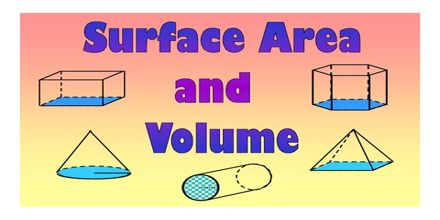Mathematic

# Lecture on Surface Area and VolumeBasic purpose of this lecture is to present on  Surface Area and Volume. Volume and surface area help us measure the size of 3D objects. We’ll start with the volume and surface area of rectangular prisms. Here briefly describe some terms like: Cube:- A solid three-dimensional geometric figure bounded by six planes; each of the six sides or faces of a cube is ∝. Cuboid:- A three-dimensional geometric figure formed of six rectangular plane faces, each set at right angles to the four sides adjacent to it. Cylinder: Cylinder consists of two circular bases of equal area in parallel planes, that are connected by a lateral surface that intersects the boundaries of the bases.